# Electric power

Electric power is defined as the rate at which electrical energy is transferred by an electric circuit. When electric current flows through a circuit with resistance, it performs "work." Various devices convert this work into many useful forms, such as heat (electric heaters), light (light bulbs), motion (electric motors), and sound (loudspeaker).

## Contents

Electricity can be produced by generation or from storage such as batteries. The SI unit of power is the watt.

## Mathematics of electric power

### In circuits

Electric power, like mechanical power, is represented by the letter P in electrical equations. The term wattage is used colloquially to mean "electric power in watts."

In direct current resistive circuits, instantaneous electrical power is calculated using Joule's Law, which is named after the British physicist James Joule, who first showed that heat and mechanical energy were interchangeable.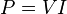$P = V I \,$

where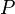$P$ is the power (watt or W)$V$ is the potential difference (volt or V)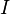$I$ is the current (ampere or A)

For example: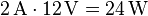$2\,\mbox{A} \cdot 12\,\mbox{V} = 24\,\mbox{W} \,$.

Joule's law can be combined with Ohm's law to produce two more equations: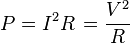$P = I^2 R\, = \frac{V^2}{R} \,$

where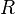$R$ is the resistance (Ohm or Ω).

For example: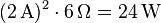$(2\,\mbox{A})^2 \cdot 6\,\Omega = 24\,\mbox{W} \,$

and$\frac{(12\,\mbox{V)}^2}{6\,\Omega} = 24\,\mbox{W} \,$

In alternating current circuits, energy storage elements such as inductance and capacitance may result in periodic reversals of the direction of energy flow. The portion of power flow that, averaged over a complete cycle of the AC waveform, results in net transfer of energy in one direction is known as real power (also referred to as active power). That portion of power flow due to stored energy, that returns to the source in each cycle, is known as reactive power.

The relationship between real power, reactive power and apparent power can be expressed by representing the quantities as vectors. Real power is represented as a horizontal vector and reactive power is represented as a vertical vector. The apparent power vector is the hypotenuse of a right triangle formed by connecting the real and reactive power vectors. This representation is often called the power triangle. Using the Pythagorean Theorem, the relationship among real, reactive and apparent power is: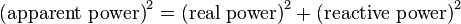$\mbox{(apparent power)}^2 = \mbox{(real power)}^2 + \mbox{(reactive power)}^2$

Real and reactive powers can also be calculated directly from the apparent power, when the current and voltage are both sinusoids with a known phase angle between them: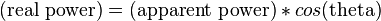$\mbox{(real power)} = \mbox {(apparent power)} * cos \mbox {(theta)}$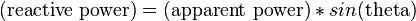$\mbox{(reactive power)} = \mbox {(apparent power)} * sin \mbox {(theta)}$

The ratio of real power to apparent power is called power factor and is a number always between zero and one.

### In space

Electrical power flows wherever electric and magnetic fields exist in the same place. The simplest example of this is in electrical circuits, as the preceding section showed. In the general case, however, the simple equation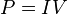$P=IV$ must be replaced by a more complex calculation, the integral of the vector cross-product of the electrical and magnetic fields over a specified area, thus: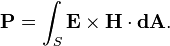$\mathbf{P} = \int_S \mathbf{E} \times \mathbf{H} \cdot \mathbf{dA}. \,$

The result is a scalar since it is the surface integral of the Poynting vector.